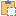#### BoundarySegment Class

An object that represents a segment of an area boundary.

Namespace: Autodesk.Revit.DB
Assembly: RevitAPI (in RevitAPI.dll) Version: 2015.0.0.0 (2015.0.0.0)

# Syntax

C#
``public class BoundarySegment : APIObject``
Visual Basic
``````Public Class BoundarySegment _
Inherits APIObject``````
Visual C++
``public ref class BoundarySegment : public APIObject``

# Remarks

These objects define the exterior boundary of an area. The geometry of the segment can be retrieved along with the element that is responsible for producing that boundary.

# ExamplesCopy VB.NET
``````Public Sub GetInfo_BoundarySegment(room As Room)
Dim segments As IList(Of IList(Of Autodesk.Revit.DB.BoundarySegment)) = room.GetBoundarySegments(New SpatialElementBoundaryOptions())

If segments IsNot Nothing Then
'the room may not be bound
Dim message As String = "BoundarySegment"
For Each segmentList As IList(Of Autodesk.Revit.DB.BoundarySegment) In segments
For Each boundarySegment As Autodesk.Revit.DB.BoundarySegment In segmentList

' Get curve start point
message += ((vbLf & "Curve start point: (" + boundarySegment.Curve.GetEndPoint(0).X & ",") + boundarySegment.Curve.GetEndPoint(0).Y & ",") + boundarySegment.Curve.GetEndPoint(0).Z & ")"
' Get curve end point
message += ((";" & vbLf & "Curve end point: (" + boundarySegment.Curve.GetEndPoint(1).X & ",") + boundarySegment.Curve.GetEndPoint(1).Y & ",") + boundarySegment.Curve.GetEndPoint(1).Z & ")"
' Get document path name
message += ";" & vbLf & "Document path name: " + boundarySegment.Document.PathName
' Get boundary segment element name
If boundarySegment.Element IsNot Nothing Then
message += ";" & vbLf & "Element name: " + boundarySegment.Element.Name
End If
Next
Next